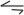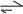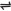## Reversible Reactions

Chemical reactions can broadly be divided into two groups: Direct reactions and reversible reactions.

Direct reactions are reactions whose reactants proceed to give the products, without the tendency of the products undergoing another reaction backward. Full arrows are used to show the direction of change in the chemical equations of direct reactions.

Example, the hypothetical equation:

aA + bB → cC + dD

A reversible reaction is one in which both the forward and backward reactions take place to a considerable extent. A reversible reaction is denoted with two half arrows pointing either sides. Example, for the hypothetical reactions:

 aA + bBcC + dD (i)
 aA + bBcC + dD (ii)
 aA + bBcC + dD (iii)

Notice that the arrows in equations (i) and (ii) are not of the same length.

Equation (i) indicates that the forward reaction is faster than the backward reaction; equation (ii) that the backward reaction is faster than the forward reaction. For equation (iii) the arrows are of the same length .

This means that both the forward and backward reactions occur at the same speed or rate.

 aA + bBcC + dD

The following process occurs in any reversible reaction.

Example, for the hypothetical one above: When substances A and B react, they produce substances C and D (forward reaction), and if the condition of reaction is suitable, C and D will in turn combine to give A and B (backward reaction).

C and D are produced at a rate proportional to the active masses of A and B (whose concentrations are initially very high but gradually decrease as C and D are formed). C and D will in turn produce A and B at a rate proportional to the active masses of C and D (whose concentrations are initially very low but gradually increase).

Hence, at the initial stage of the reaction, the forward reaction will be much faster. As the reaction progresses, the concentration of A and B will fall while those of C and D will rise, until gradually the inequality between the rates of the opposing reactions cancel out.

At this point, the state of chemical equilibrium have been attained - the reaction will proceed in either direction at exactly the same rate.

Once equilibrium condition has been attained, there is no change in the concentrations of the substances in the reaction mixture because then, products of each reaction (forward and backward) are being used up as fast as they are being formed simultaneously.

Hence, the equilibrium condition can be described as dynamic rather than static - that is, it is an equilibrium involving the constant interchange of particles in motion, the rate of both forward and backward reactions are exactly equal.

Note: dynamic equilibrium is only possible in a closed system - i.e. if there is no removal or addition of any of the materials in the system.

Examples of reversible reactions:

(1). Thermal dissociation of dinitrogen tetraoxide:

 N2O4(g)2NO2(g)

(2). Action of steam on red hot iron:

 3Fe(s) + 4H2O(g)Fe3O4(s) + 4H2(g)

(3). Oxidation of sulphur(IV) oxide:

 2SO2(g) + O2(g)2SO3(g)

(4). Synthesis of ammonia:

 N2(g) + 3H2(g)2NH3(g)

Characteristics of Equilibrium Processes

1. No external aid is required to move a chemical system to equilibrium state - it is a spontaneous change. But a system already in equilibrium will only change by the effect of an external influence (e.g. change in temperature and pressure).

2. The equilibrium state is dynamic - i.e., the rate of both forward and backward reactions are exactly equal.

3. Irrespective of the direction from which it is reached, equilibrium position is the same.

4. The equilibrium state is a balance of two opposing tendencies - the drive for molecules to reach the state of minimum energy, and the drive towards a state of maximum entropy.

Related Posts

 Like This Post? Please Share!!!!!!!

Copyright , All Rights Reserved Free Chemistry Online | About Us | Usage of Content | Total Disclosures | Privacy Policy